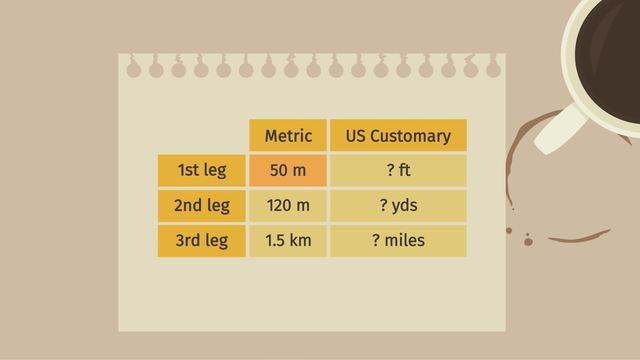# Getting the Job Done: Converting Units of MeasurementRating

Be the first to give a rating!

The authorsChris S.

## Basics on the topicGetting the Job Done: Converting Units of Measurement

After this lesson, you will be able to use rates, ratios, and the conversation factor, to convert units of measurement.

The lesson begins by reminding you how to set up a ratio table with equivalent ratios, then use that to set up a proportion. It then teaches you how to use multiplication by the conversion factor, written as a fraction, to convert units.

Learn how to convert units of measurement by helping Maxwell send the rover on a mission to Mars!

This video includes key concepts, notation, and vocabulary such as: a ratio table (a table of equivalent ratios); conversion factor (a unit rate written as a fraction, used to change one unit of measurement to another); and unit rates (a comparison of two measurements with one of the measurements equal to 1).

Before watching this video, you should already be familiar with ratio tables, equivalent ratios, unit rates, and conversion tables.

After watching this video, you will be prepared to learn more about the relationship between ratios, rates, and converting units.

Common Core Standard(s) in focus: 6.RP.A.3.d A video intended for math students in the 6th grade Recommended for students who are 11-12 years old